## Example Questions

### Example Question #1 : Polynomials

If 3 less than 15 is equal to 2x, then 24/x must be greater than

4

6

3

5

3

Explanation:

Set up an equation for the sentence: 15 – 3 = 2x and solve for x.  X equals 6. If you plug in 6 for x in the expression 24/x, you get 24/6 = 4. 4 is only choice greater than a.

### Example Question #2 : Polynomial Operations

Given a♦b = (a+b)/(a-b) and b♦a = (b+a)/(b-a), which of the following statement(s) is(are) true:

I. a♦b = -(b♦a)

II. (a♦b)(b♦a) = (a♦b)2

III. a♦b + b♦a = 0

II & III

I only

I and II

I, II and III

I and III

I and III

Explanation:

Notice that - (a-b) = b-a, so statement I & III are true after substituting the expression. Substitute the expression for statement II gives ((a+b)/(a-b))((a+b)/(b-a))=((a+b)(b+a))/((-1)(a-b)(a-b))=-1 〖(a+b)〗2/〖(a-b)〗2 =-((a+b)/(a-b))2 = -(a♦b)2 ≠ (a♦b)2

### Example Question #1 : Polynomials

If a positive integer a is divided by 7, the remainder is 4.  What is the remainder if 3a + 5 is divided by 3?

4

5

6

2

3

2

Explanation:

The best way to solve this problem is to plug in an appropriate value for a.  For example, plug-in 11 for because 11 divided by 7 will give us a remainder of 4.

Then 3a + 5, where = 11, gives us 38.  Then 38 divided by 3 gives a remainder of 2.

The algebra method is as follows:

a divided by 7 gives us some positive integer b, with a remainder of 4.

Thus,

/ 7 = b  4/7

/ 7 = (7b + 4) / 7

a = (7b + 4)

then 3a + 5 = 3 (7+ 4) + 5

(3a+5)/3 = [3(7+ 4) + 5] / 3

= (7+ 4) + 5/3

The first half of this expression (7b + 4) is a positive integer, but the second half of this expression (5/3) gives us a remainder of 2.

### Example Question #1 : Polynomials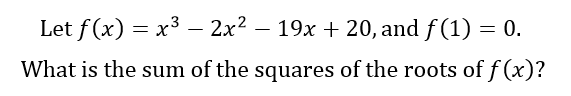36

38

100

45

42

42

Explanation: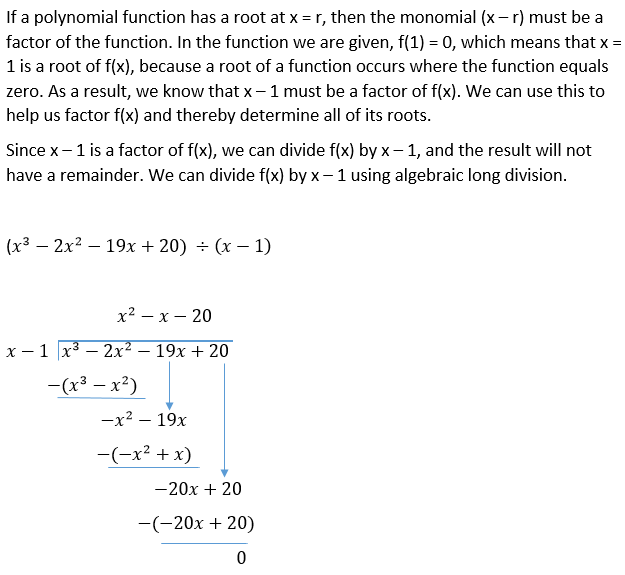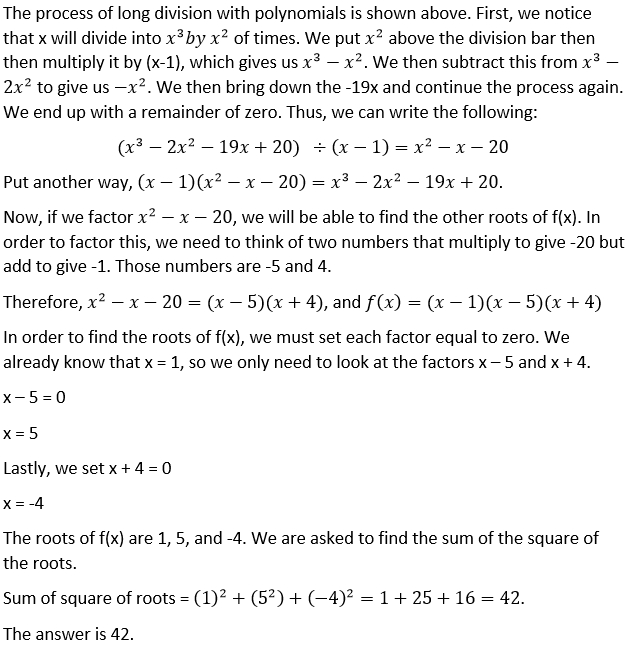### Example Question #2151 : Sat Mathematics

Simplify: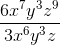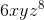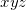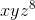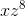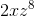Explanation:

Cancel by subtracting the exponents of like terms: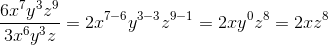### Example Question #2152 : Sat Mathematics

By what expression can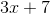be multiplied to yield the product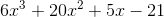?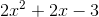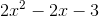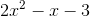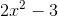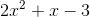Explanation:

Dividebyby setting up a long division.

Divide the lead term of the dividend,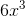, by that of the divisor,; the result is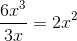Enter that as the first term of the quotient. Multiply this by the divisor: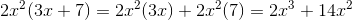Subtract this from the dividend. This is shown in the figure below.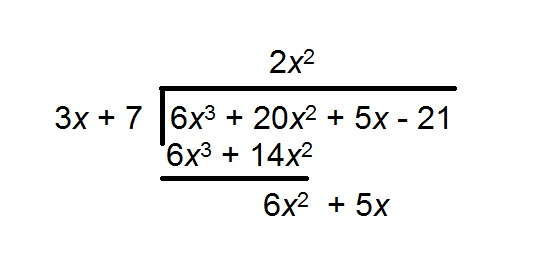Repeat the process with the new difference: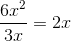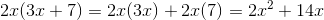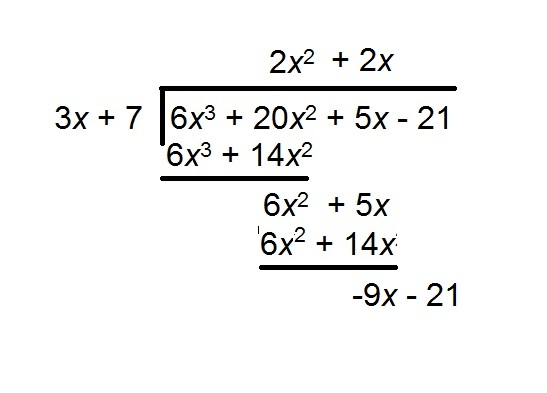Repeating: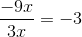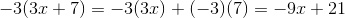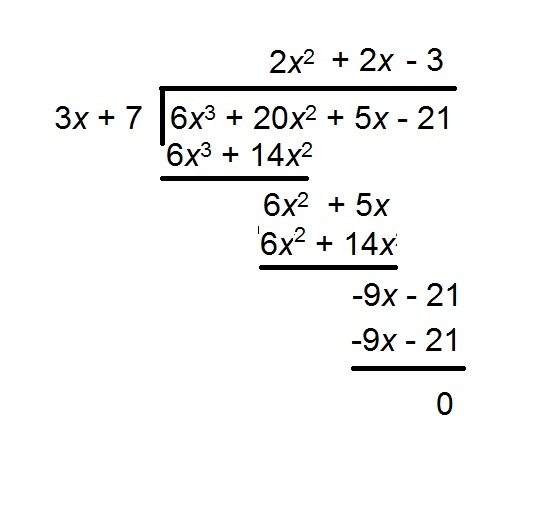The quotient - and the correct response - is.

### Example Question #371 : Algebra

Divide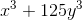by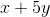.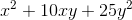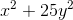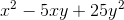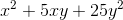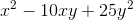Explanation:

It is not necessary to work a long division if you recognizeas the sum of two perfect cube expressions: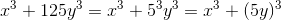A sum of cubes can be factored according to the pattern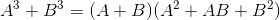,

so, setting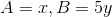,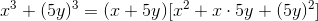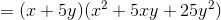Therefore,### All SAT Math Resources